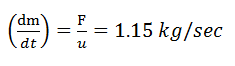# If the force on a rocket moving with a velocity of 300 m/sec is 345N, then the rate of combustion of the fuel, is         (a)    0.55 kg/sec         (b)    0.75 kg/sec         (c)    1.15 kg/sec        (d)    2.25 kg/secNavjyot Kalra
10 years ago

(c)
Velocity of the rocket (u) = 300 m/s and force (F) = 345N.
Rate of combustion of fuel
(dm/dt)=F/u=1.15 kg/sec

10 years ago

(c)

Velocity of the rocket (u) = 300 m/s and force (F) = 345N.

Rate of combustion of fuel